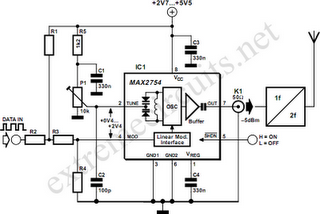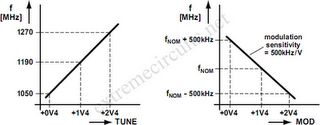# Learning Electronics

Learn to build electronic circuits

# 1.2GHz VCO With Linear Modulation

Since high frequency voltage-controlled oscillators, or VCOs, are not easy to construct, Maxim (www.maxim-ic.com) has produced an integrated 1.2GHz oscillator, the MAX2754. The center frequency is set using the TUNE input, and a linear modulation input allows the frequency to be modulated. The IC is available in an 8-pin µMAX package, operates from a supply of between 2.7 V and 5.5 V, and draws a current of less than 2 mA. Both TUNE and MOD operate over control voltage range of +0.4 V to +2.4 V. TUNE allows the VCO frequency to be adjusted from 1050 MHz to 1270 MHz. In some applications a PLL control voltage will be applied here, allowing the center frequency to be set exactly to a desired value.For simplicity in the circuit diagram we have shown a potentiometer. The MOD input allows the VCO to be modulated in a digital or analogue fashion, with a transfer slope of –500 kHz/V. In the circuit we have shown an example where MOD is used for frequency shift keying (FSK) modulation. Resistors R1 to R4 shift the level of the data signal so that it has a center value of +1.4 V and an amplitude corresponding to the desired frequency deviation. One example set of values, suitable for use with a 5 V power supply, is as follows: R1 = 480 Ω, R2 = 100 Ω, R3 = 220 Ω und R4 = 270 Ω.The input impedance is about 1 kΩ. The output level of the MAX2754 at OUT is around –5dBm into 50 Ω. A coupling capacitor is not required here: the IC already contains one. The MAX2754 is designed for use in transmitters in the 2.4GHz ISM (industrial, scientific and medical) band. This requires the addition of a frequency doubler, which, along with the 2.4GHz antenna, is shown symbolically in the circuit diagram.